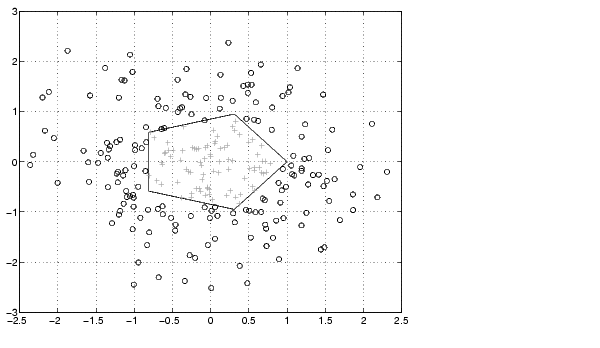MATLAB Function Referenceinpolygon

Detect points inside a polygonal region

Syntax

• ```IN = inpolygon(X,Y,xv,yv)
[IN ON] = inpolygon(X,Y,xv,yv)
```

Description

```IN = inpolygon(X,Y,xv,yv) ``` returns a matrix `IN` the same size as `X` and `Y`. Each element of `IN` is assigned the value 1 or 0 depending on whether the point `(X(p,q),Y(p,q))` is inside the polygonal region whose vertices are specified by the vectors `xv` and `yv`. In particular:

 `IN(p,q) = 1` If `(X(p,q),Y(p,q))` is inside the polygonal region or on the polygon boundary `IN(p,q) = 0` If `(X(p,q),Y(p,q))` is outside the polygonal region

```[IN ON] = inpolygon(X,Y,xv,yv) ``` returns a second matrix `ON` the same size as `X` and `Y`. Each element of `ON` is assigned the value 1 or 0 depending on whether the point `(X(p,q),Y(p,q))` is on the boundary of the polygonal region whose vertices are specified by the vectors `xv` and `yv`. In particular:

 `IN(p,q) = 1` If `(X(p,q),Y(p,q))` is on the polygon boundary `IN(p,q) = 0` If `(X(p,q),Y(p,q))` is inside or outside the polygon boundary

Examples

• ```L = linspace(0,2.*pi,6); xv = cos(L)';yv = sin(L)';
xv = [xv ; xv(1)]; yv = [yv ; yv(1)];
x = randn(250,1); y = randn(250,1);
in = inpolygon(x,y,xv,yv);
plot(xv,yv,x(in),y(in),'r+',x(~in),y(~in),'bo')```

© 1994-2005 The MathWorks, Inc.